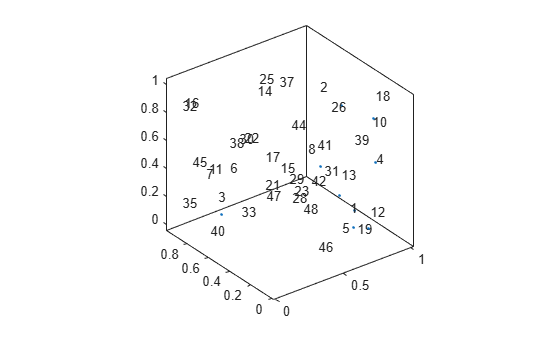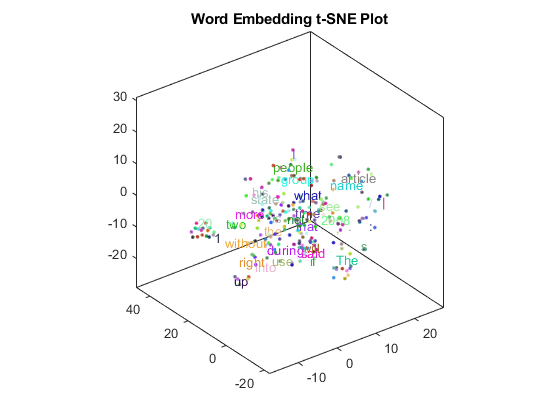# textscatter3

3-D scatter plot of text

## Syntax

``ts = textscatter3(x,y,z,str)``
``ts = textscatter3(xyz,str)``
``ts = textscatter3(ax,___)``
``ts = textscatter3(___,Name,Value)``

## Description

example

````ts = textscatter3(x,y,z,str)` creates a 3-D text scatter plot with elements of `str` at the locations specified by the vectors `x`, `y`, and `z`.```

example

````ts = textscatter3(xyz,str)` creates a 3-D text scatter plot with elements of `str` at the locations specified by the rows of `xyz`. This syntax is equivalent to `textscatter(xyz(:,1),xyz(:,2),xyz(:,3),str)`.```
````ts = textscatter3(ax,___)` plots into axes object `ax`. Use this syntax with any of the input arguments in previous syntaxes.```

example

````ts = textscatter3(___,Name,Value)` specifies additional `TextScatter` properties using one or more name-value pair arguments.```

## Examples

collapse all

Plot a string array of numbers at random points on a 3-D text scatter plot.

```x = rand(50,1); y = rand(50,1); z = rand(50,1); str = string(1:50); figure textscatter3(x,y,z,str);```Alternatively, you can pass the coordinates `x`, `y`, and `z` as a matrix `xyz`, where `x`, `y`, and `z` are the columns of `xyz`.

```xyz = [x y z]; figure textscatter3(xyz,str)```Create text scatter plot of a word embedding and specify word colors.

Load a pretrained word embedding using `fastTextWordEmbedding`. This function requires Text Analytics Toolbox™ Model for fastText English 16 Billion Token Word Embedding support package. If this support package is not installed, then the function provides a download link.

`emb = fastTextWordEmbedding;`

Convert the first 250 words to vectors using `word2vec`. `V` is a matrix of word vectors of length 300.

```words = emb.Vocabulary(1:250); V = word2vec(emb,words); size(V)```
```ans = 1×2 250 300 ```

Embed the word vectors in a 3-D space using `tsne`.

`XYZ = tsne(V,'NumDimensions',3);`

Plot the words at the coordinates specified by `XYZ` in a 3-D text scatter plot. Specify the word colors to be random.

```numWords = numel(words); colorData = rand(numWords,3); figure textscatter3(XYZ,words,'ColorData',colorData) title("Word Embedding t-SNE Plot")```## Input Arguments

collapse all

x values, specified as a vector. `x`, `y`, `z`, and `str` must be of equal length.

Example: `[1 2 3]`

y values, specified as a vector. `x`, `y`, `z`, and `str` must be of equal length.

Example: `[1 2 3]`

z values, specified as a vector. `x`, `y`, `z`, and `str` must be of equal length.

Example: `[1 2 3]`

x, y, and z values, specified as a matrix. The first, second, and third columns of `xyz` correspond to the x, y, and z values, respectively.

Input text, specified as a string array or cell array of character vectors. `x`, `y`, `z`, and `str` must be of equal length.

Example: `["one" "two" "three"]`

Data Types: `string` | `cell`

Axes object. If you do not specify an axes object, then the function uses the current axes.

### Name-Value Arguments

Specify optional pairs of arguments as `Name1=Value1,...,NameN=ValueN`, where `Name` is the argument name and `Value` is the corresponding value. Name-value arguments must appear after other arguments, but the order of the pairs does not matter.

Before R2021a, use commas to separate each name and value, and enclose `Name` in quotes.

Example: `'Marker','*'` specifies the markers to be asterisks.

The `TextScatter` object properties listed here are only a subset. For a complete list, see TextScatter Properties.

Percentage of text data to show, specified as a scalar from 0 through 100. To show all text, set `TextDensityPercentage` to 100. To show no text, set `TextDensityPercentage` to 0.

If you set `TextDensityPercentage` to 100, then the software does not plot markers.

Example: 70

Maximum length of text labels, specified as a positive integer. The software truncates the text labels to this length and adds ellipses at the point of truncation.

Example: 10

Marker colors, specified as one of these values:

• `'auto'` — For each marker, use the same color as the corresponding text labels.

• `'none'` — Do not show markers.

• RGB triplet — Use the same color for all the markers in the plot. An RGB triplet is a three-element row vector whose elements specify the intensities of the red, green, and blue components of the color. The intensities must be in the range `[0,1]`; for example, ```[0.5 0.6 0.7]```.

Example: `[1 0 0]`

Text colors, specified as one of these values:

• RGB triplet — Use the same color for all the text in the plot. An RGB triplet is a three-element row vector whose elements specify the intensities of the red, green, and blue components of the color. The intensities must be in the range `[0,1]`; for example, ```[0.5 0.6 0.7]```.

• Three-column matrix of RGB triplets — Use a different color for each text label in the plot. Each row of the matrix defines one color. The number of rows must equal the number of text labels.

• Categorical vector — Use a different color for each category in the vector. Specify `ColorData` as a vector the same length as `XData`. Specify the colors for each category using the `Colors` property

Example: `[1 0 0; 0 1 0; 0 0 1]`

Category colors, specified as a matrix of RGB triplets. An RGB triplet is a three-element row vector whose elements specify the intensities of the red, green, and blue components of the color. The intensities must be in the range `[0,1]`; for example, `[0.5 0.6 0.7]`.

By default, `Colors` is equal to the `ColorOrder` property of the axes object.

Example: `[1 0 0; 0 1 0; 0 0 1]`

## Output Arguments

collapse all

`TextScatter` object. Use `ts` to access and modify properties of the text scatter chart after it has been created. For more information, see TextScatter Properties.

## Version History

Introduced in R2017b